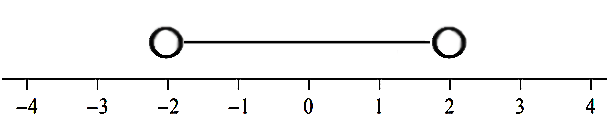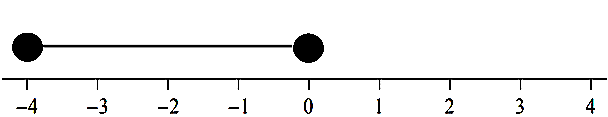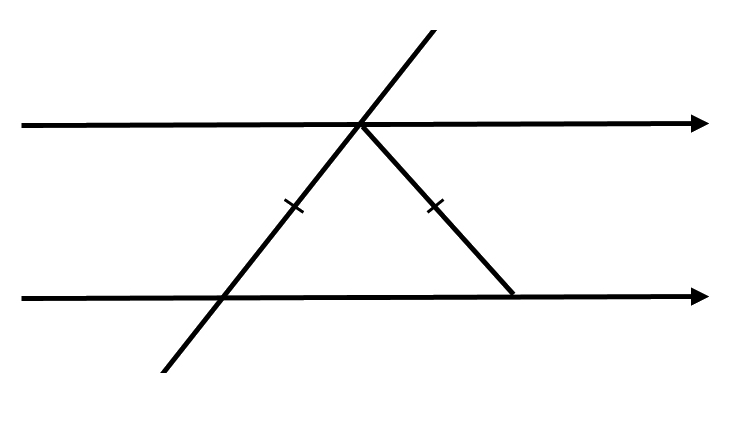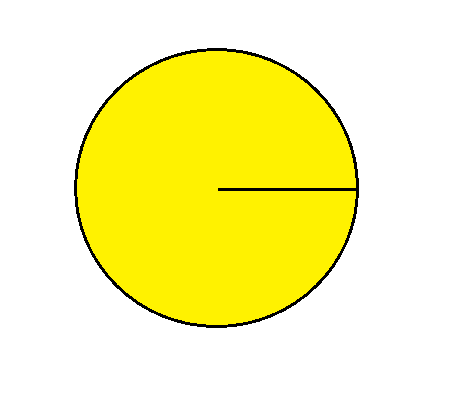You need to solve a linear equation, try expanding the bracket first.Click to visit the maths genie
Dividing fractions. You must do something to the second fraction first... Click to visit the maths genie
This is a mixed skill number problem. You will need to be able to find a fraction of an amount and a percentage of an amount.
Solving a linear equation, start by getting the xs on to one side.
This question is about finding the area of a trapezium. 1/2(a+b)h. Click to visit the maths genie
Parallel lines - You need to know your corresponding angles from your alternate angles... Click to visit the maths genie
This is about inequalities. Click to visit the maths genie
This is a ratio question where one amount has been given, but not the total amount. Drawing a bar model might help. Click to visit the maths genie
This is about sharing amounts in ratio. Begin by working out how many parts are in the ratio altogether. Click to visit the maths genie
This is about areas of circle. Does the formula Pi r^2 ring any bells for you? If not then click to visit the maths genie
This is about areas of circle. Does the formula Pi d ring any bells for you? Or 2 pi r ? If not then click to visit the maths genie
Coming soon
Coming soon